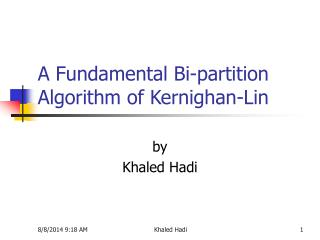DownloadDownload PresentationA Fundamental Bi-partition Algorithm of Kernighan-Lin

# A Fundamental Bi-partition Algorithm of Kernighan-Lin

Download Presentation## A Fundamental Bi-partition Algorithm of Kernighan-Lin

- - - - - - - - - - - - - - - - - - - - - - - - - - - E N D - - - - - - - - - - - - - - - - - - - - - - - - - - -
##### Presentation Transcript

1. A Fundamental Bi-partition Algorithm of Kernighan-Lin by Khaled Hadi Khaled Hadi

2. Outline • Introduction • Problem Definition • Kernighan-Lin Algorithm (K-L Algorithm) • K-L Algorithm : A Simple Example • K-L Algorithm : A Weighted Example • Time Complexity • Drawbacks of the K-L Heuristic and Conclusion Khaled Hadi

4. Levels of Partitioning • The levels of partitioning: system, board, chip. • Hierarchical partitioning: higher costs for higher levels. Khaled Hadi

5. Circuit Partitioning • Objective: Partition a circuit into parts such that every component is within a prescribed range and the # of connections among the components is minimized. • More constraints are possible for some applications. • Cutset? Cut size? Size of a component? Khaled Hadi

6. Problem Definition: Partitioning • k-way partitioning: Given a graph G(V, E), where each vertex v belongs toV has a size s(v) and each edge e belongs to E has a weight w(e), the problem is to divide the set V into k disjoint subsets V1, V2, …, Vk, such that an objective function is optimized, subject to certain constraints. • Bounded size constraint: The size of the i-th subset is bounded by • Min-cut cost between two subsets: Minimize , where p(u) is the partition # of node u. • The 2-way, balanced partitioning problem is NP-complete, even in its simple form with identical vertex sizes and unit edge weights. Khaled Hadi

7. Kernighan-Lin Algorithm • Kernighan and Lin, “An efficient heuristic procedure for partitioning graphs,” The Bell System Technical Journal, vol. 49, no. 2, Feb. 1970. • An iterative, 2-way, balanced partitioning (bi-sectioning) heuristic. • Till the cut size keeps decreasing • Vertex pairs which give the largest decrease or smallest increase in cut size are exchanged. • These vertices are then locked (and thus are prohibited from participating in any further exchanges). • This process continues until all the vertices are locked. • Find the set with the largest partial sum for swapping. • Unlock all vertices. Khaled Hadi

8. Kernighan-Lin Algorithm: A Simple Example • Each edge has a unit weight. Questions: How to compute cost reduction? What pairs to be swapped? Consider the change of internal & external connections. Khaled Hadi

11. Kernighan-Lin Algorithm: A Weighted Example • Iteration 1: Khaled Hadi

12. A Weighted Example (cont’d) Iteration 1: Khaled Hadi

13. A Weighted Example (cont’d) Khaled Hadi

14. A Weighted Example (cont’d) Khaled Hadi

15. A Weighted Example (cont’d) Khaled Hadi